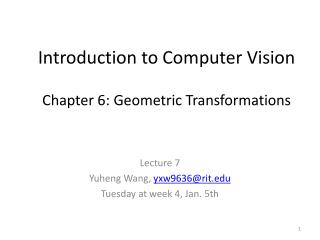DownloadDownload PresentationIntroduction to Computer Vision Chapter 6: Geometric Transformations

# Introduction to Computer Vision Chapter 6: Geometric Transformations

Download Presentation## Introduction to Computer Vision Chapter 6: Geometric Transformations

- - - - - - - - - - - - - - - - - - - - - - - - - - - E N D - - - - - - - - - - - - - - - - - - - - - - - - - - -
##### Presentation Transcript

1. Introduction to Computer VisionChapter 6: Geometric Transformations Lecture 7 Yuheng Wang, yxw9636@rit.edu Tuesday at week 4, Jan. 5th

2. Outline • Geometric Transformation Theory • Linear transformations • Affine transformations • Projective transformations • Matlab Implementation

3. Introduction • Modify spatial relationships between

4. Geometric Transformations • Original Image

5. Geometric Transformations • Scaling

6. Geometric Transformations • Rotation

7. Geometric Transformations • Affine Transformation

8. Geometric Transformations • Projective Transformation

9. Coordinate Mapping • Transformation: (x, y) = T{(w, z)} T(w, z) y z x w f(w, z) g(x, y )

10. Coordinate Mapping • Transformation: (w, z) = T-1{(x, y)} T-1 (x, y) z y w x f(w, z) g(x, y )

11. (w, z)= (2x, 2y) Textbook Page 280 Figure 6.2 (x, y)= (w/2,z/2) Transformations: (x, y) = T{(w, z)} = (w/2,z/2) (w, z) = T-1{(x, y)} = (2x, 2y)

12. Linear Transformations • An operation than change the figure in some way, can be either or a combination of: • Translation: “slide” • Rotation: “turn” • Reflection: “flip” • Reference: • http://www.cs.mtu.edu/~shene/COURSES/cs3621/NOTES/geometry//geo-tran.html

13. Translation • Move the object without changing the image size and orientation

14. Rotation • Turn the object about around certain fixed point (the rotation center) Rotation Center

15. Reflection • Flip the object over a line (the line of reflection) Line of Reflection

16. Reflection

17. Method to implement transformations Find coordinates (x, y) of the original image Use the transformation rule to change original coordinates (x, y) to (x’, y’) Show the new image using (x’, y’)

18. Translation x’= x + 4 y’= y - 1 y 0 x

19. Rotation theta = 90o y 0 x

20. Reflection y 0 x

21. Scaling y 0 x

22. Scaling • multiplying each of its • coordinates by a scalar y x 0

23. Overall workflow Find coordinates (x, y) of the original image Use the transformation rule to change original coordinates (x, y) to (x’, y’) Show the new image using (x’, y’)

24. Mathematical Definition • A geometric transformation is a matrix(vector) T that maps the pixel (x, y) to a new position (x’, y’) x’ = Tx(x, y) y’=Ty(x, y)

25. Linear Transformations • Recall: • x’ = Tx(x, y) y’=Ty(x, y) • Polynomial Format • Matrix Format Transformation Matrix T

26. Affine Transformations • Recall: • x’ = Tx(x, y) y’=Ty(x, y) • Polynomial Format • Matrix Format Transformation Matrix T

27. Affine Transformations • Perceptually, Affine Transformations are linear combinations of • Translation • Rotation • Scaling

28. LinearTransformations y • Rotation x 0

29. LinearTransformations y • Scaling x 0

30. LinearTransformations y • Reflection along X-axis x 0

31. LinearTransformations • Reflection along Y-axis y x 0

32. Linear Transformations • Shearing along X-axis y x 0

33. LinearTransformations • Shearing along Y-axis y x 0

34. AffineTransformations y • Rotation x 0

35. Affine Transformations • Scaling y x 0

36. Affine Transformations y • Shearing x 0

37. Affine Transformations y • Reflection x 0

38. Affine Transformations y • Translation x 0

39. Summary Translation Scaling Rotation Shearing

40. Textbook Page 286 Table 6.1 Summary

41. Projective Transformations

42. Vanishing Point Horizontal Line Vanishing Point

43. Matlab Implementation • Transformation Techniques • Affine Transformations • Projective Transformations • Matlab Implementation • function maketform • function imtransform

44. help maketform • maketform Create spatial transformation structure (TFORM). • T = maketform(TRANSFORMTYPE,...) creates a multidimensional spatial transformation structure (a 'TFORM struct') that can be used with TFORMFWD, TFORMINV, FLIPTFORM, IMTRANSFORM, or TFORMARRAY. TRANSFORMTYPE can be 'affine', 'projective', 'custom', 'box', or 'composite'. Spatial transformations are also called geometric transformations. • ……

45. Several Forms • T = maketform('affine',A); • T = maketform('affine',U,X); • T = maketform('projective',A); • T = maketform('projective',U,X); • T = maketform('custom',NDIMS_IN,NDIMS_OUT, FORWARD_FCN,INVERSE_FCN, TDATA);

46. T = maketform('custom',NDIMS_IN, NDIMS_OUT,FORWARD_FCN,INVERSE_FCN, TDATA • Parameters • NDIMS_IN and NDIMS_OUT are the numbers of input and output dimensions. • FORWARD_FCN and INVERSE_FCN are function handles to forward and inverse functions. • TDATA can be any MATLAB array and is typically used to store parameters of the custom transformation. It is accessible to FORWARD_FCN and INVERSE_FNC via the "tdata" field of T.

47. help imtransform • imtransform Apply 2-D spatial transformation to image. • B = imtransform(A,TFORM) transforms the image A according to the 2-D spatial transformation defined by TFORM, which is a tform structure as returned by MAKETFORM or CP2TFORM. If ndims(A) > 2, such as for an RGB image, then the same 2-D transformation is automatically applied to all 2-D planes along the higher dimensions. • ……

48. clear all, close all, clc Im = checkerboard(50); figure, imshow(Im), axis on; title('Original Checkerboard');

49. Im = imread('cameraman.tif');figure, imshow(Im), axis on;title(‘Fig.1. Original Gray Scale Image'); axis on

50. Matlab for Scaling sx=0.5; sy=1; Tscale=[sx 0 0; 0 sy 0; 0 0 1]; tScale=maketform('affine',Tscale); ImScale=imtransform(Im, tScale); figure, imshow(ImScale), axis on; title('Scaled Checkerboard'); Textbook Page 289